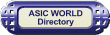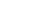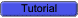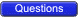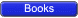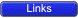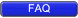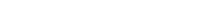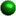Logic CircuitsBoolean algebra is ideal for expressing the behavior of logic circuits.A circuit can be expressed as a logic design and implemented as a collection of individual connected logic gates.Fixed Logic Systems

A fixed logic system has two possible choices for representing true and false.Positive Logic

In a positive logic system, a high voltage is used to represent logical true (1), and a low voltage for a logical false (0).Negative Logic

In a negative logic system, a low voltage is used to represent logical true (1), and a high voltage for a logical false (0).In positive logic circuits it is normal to use +5V for true and 0V for false.Switching Circuits

The abstract logic described previously can be implemented as an actual circuit. Switches are left open for logic 0 and closed for logic 1.Two variable AND circuit X.Y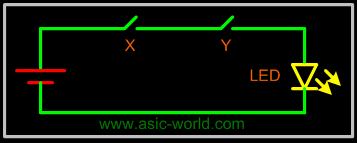Two variable OR circuit X + Y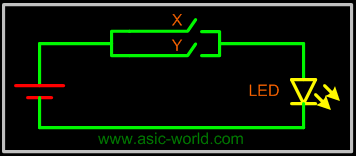Four variable circuit U.V.(X + Y)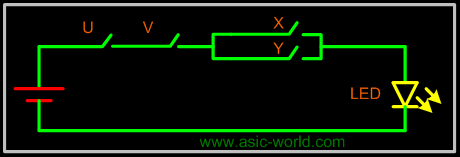Truth Table

A truth table is a means for describing how a logic circuit's output depends on the logic levels present at the circuit's inputs.In the following twos-inputs logic circuit, the table lists all possible combinations of logic levels present at inputs X and Y along with the corresponding output level F.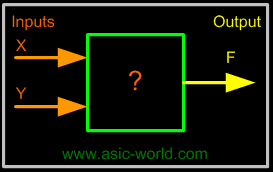X Y F = X*Y 0 0 0 0 1 0 1 0 0 1 1 1When either input X AND Y is 1, the output F is 1. Therefore the "?" in the box is an AND gate.Copyright © 1998-2014 Deepak Kumar Tala - All rights reserved Do you have any Comment? mail me at:deepak@asic-world.com